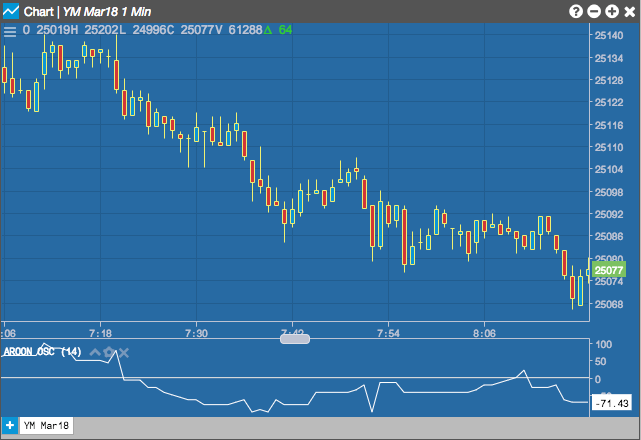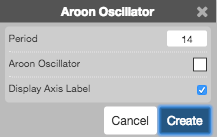Charts

# Aroon Oscillator (ARO)

The Aroon Oscillator is a derivative of the Aroon indicator that provides a different way to view the results. It is constructed by subtracting Aroon Down from Aroon Up. The Aroon Oscillator oscillates between -100 and +100 with zero as the center line.

The Aroon Oscillator provides the following signals:

• Above zero signals an uptrend.
• Below zero indicates a downtrend.## Configuration Options• Field: Price or combination of prices to use as the base for average calculations. Possible values include:
• Open
• High
• Low
• Close
• HL/2 $$\left ( \frac{High + Low}{2} \right )$$
• HLC/3 $$\left ( \frac{High + Low + Close}{3} \right )$$
• HLCC/4 $$\left ( \frac{High + Low + Close + Close}{4} \right )$$
• OHLC/4 $$\left ( \frac{Open + High + Low + Close}{4} \right )$$
• Color Selectors: Colors to use for graph elements.
• Display Axis Label: Whether to display the most recent value on the Y axis.

## Formula

$Aroon\;Osc = Aroon\;Up - Aroon\;Down$

The formula uses the following equations, where n is the number of days:

$Aroon\;Up = \frac{100 * (n - (Days\;Since\;n-day\;High))}{n}$

$Aroon\;Down = \frac{100 * (n - (Days\;Since\;n-day\;Low))}{n}$